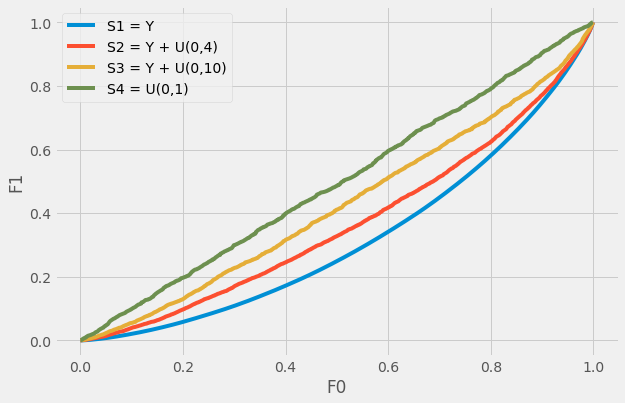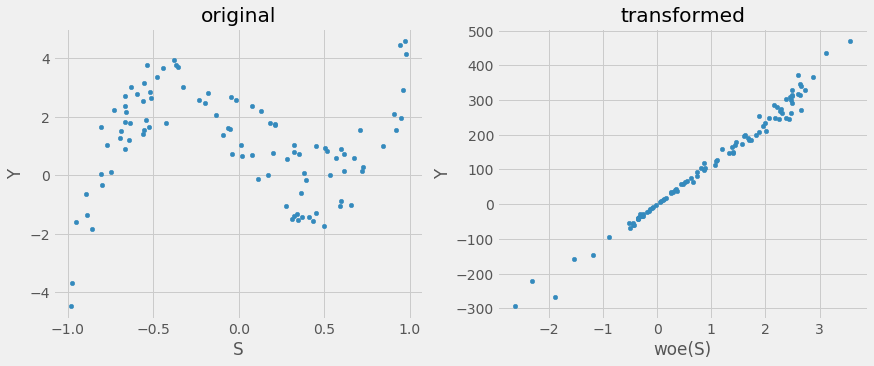# Univariate Analysis IV¶

Nov. 17, 2020 $\newcommand{\bs}{\boldsymbol}$ $\newcommand{\argmin}{\underset{\bs{#1}}{\text{arg min}}\,}$ $\newcommand{\argmax}{\underset{\bs{#1}}{\text{arg max}}\,}$ $\newcommand{\tr}{^{\top}}$ $\newcommand{\norm}{\left|\left|\,#1\,\right|\right|}$ $\newcommand{\given}{\,|\,}$ $\newcommand{\st}{\,\big|\,}$ $\newcommand{\E}{\mathbb{E}\left[#1\right]}$ $\newcommand{\P}{\mathbb{P}\left(#1\right)}$ $\newcommand{\abs}{\left|#1\right|}$ $\newcommand{\blue}{{\color{blue} {#1}}}$ $\newcommand{\red}{{\color{red} {#1}}}$ $\newcommand{\orange}{{\color{orange} {#1}}}$ $\newcommand{\pfrac}{\frac{\partial #1}{\partial #2}}$

Recently, I worked on projects where univariate analysis played a vital role in their success. My earlier attempts to generalize the theory involve breaking $Y$ into a binary variable in order to calculate metrics such as AUC and gini, but in doing this we lose information. In this post I would like to propose a better solution.

## Generalized Lorenz Curve¶

Suppose $Y$ follow a distribution from the exponential family. (E.g. the Gamma distribution.) Let $S$ be another variable that is associated with $Y$. We denote the relationship by $Y = f(S)$. Further, let $g$ denote the link function and $\beta_0, \beta_1$ are constants. We have the GLM framework:

$$g(E(Y)) = \beta_0 + \beta_1 S. \tag{1}$$

Given i.i.d. sequence $S_1,...,S_N$, and a corresponding sequence $Y_1,...,Y_N$, we define the cumulative distribution of $S$ weighted by $Y$ as

$$F_1(s) = \frac{1}{\sum_{k=1}^N Y_k} \sum_{k=1}^N Y_k I(S_k\leq s). \tag{2}$$

Remark   Equation (2) is a valid CDF only if $Y$ is nonnegative.

To show that we must check

• $F_1(s)$ is non-decreasing. This is true only if $Y$ is nonnegative.
• $F_1(s)$ is right continuous. True by the definition of the indicator function.
• $\lim_{s\to\infty} F_1(s) = 1$ and $\lim_{s\to -\infty} F_1(s) = 0$ are true clearly by definition.

In general, $F_1(s)$ measures the cumulative growth of $Y$ rank-ordered by $S$. If we replace $Y_k$ in (2) by a constant variable $C$, we have

$$F_0(s) = \frac{1}{\sum_{k=1}^N C} \sum_{k=1}^N C I(S_k \leq s) = \frac{1}{N} \sum_{k=1}^N I(S_k \leq s), \tag{3}$$

which is exactly the ordinary cumulative distribution (CDF) of $S$.

For illustration, we simulate 1000 instances the following random variables.

• $Y \sim \text{Gamma}(2,1)$
• $S^{(1)} = Y$
• $S^{(2)} = Y + \varepsilon$, where $\epsilon \sim U(0,4)$.
• $S^{(3)} = Y + \varepsilon$, where $\epsilon \sim U(0,10)$.
• $S^{(4)} \sim U(0,1)$,

where $U(a,b)$ represents random noise uniformly distributed between $a$ and $b$. Due to the nature of the simulation, equation (2) can be conveniently estimated by the sequence $0, 0.001, 0.002,...,0.999$. The cumulative sum distribution of $S^{(i)}$ can be calculated by first sorting the dataframe by $S^{(i)}$ and use the cumsum function on $Y$.

In :
import numpy as np
import pandas as pd
import matplotlib.pyplot as plt
plt.style.use('fivethirtyeight')

# generate 1000 iid samples of Y, S1, S2, S3, S4
N = 1000
np.random.seed(91)
Y = np.random.gamma(2,1,N)
S1 = Y
S2 = Y + 4*np.random.rand(N)
S3 = Y + 10*np.random.rand(N)
S4 = np.random.rand(N)

# save in a dataframe
df = pd.DataFrame(zip(Y,S1,S2,S3,S4),columns=['Y','S1','S2','S3','S4'])

# calculate F0 and F1
F0 = np.arange(0,1000,1)/1000
F11 = df.sort_values(by='S1').Y.cumsum()/df.Y.sum()
F12 = df.sort_values(by='S2').Y.cumsum()/df.Y.sum()
F13 = df.sort_values(by='S3').Y.cumsum()/df.Y.sum()
F14 = df.sort_values(by='S4').Y.cumsum()/df.Y.sum()

# plot
fig, ax = plt.subplots(figsize=(9,6))
ax.plot(F0, F11, label='S1 = Y')
ax.plot(F0, F12, label='S2 = Y + U(0,4)')
ax.plot(F0, F13, label='S3 = Y + U(0,10)')
ax.plot(F0, F14, label='S4 = U(0,1)')
ax.set_xlabel('F0'); ax.set_ylabel('F1')
plt.legend(); plt.show()This is the generalized Lorenz curve. Recall for binary target, the Lorenz curve can theoretically reach the bottom right corner of the chart. In that case, the AUC and the Gini both equal to 1. In the generalized case, the Lorenz curve can only go as "far" as $S^{(1)}$ (the blue curve). From here, it becomes clear that we can generalize all the metrics (KS, Gini, AUC, information value) similarly as before. The only caveate is that the AUC is no longer between 0 and 1. This can be solved by applying an appropriate normalization.

## Generalized Weight of Evidence¶

Let $\mathcal{S}$ and $\mathcal{Y}$ denote the support of $S$ and $Y$, respectively. If $\mathcal{S}$ is a closed interval in $\mathbb{R}$, define a partition of $\mathcal{S}$ by

$$(q_0, q_1], (q_1, q_2], \cdots, (q_{r-1}, q_{r}] \subset \mathcal{S},$$

where $q_0 = -\infty$ and $q_r = \max \mathcal{S}$.

Given $S$, define $\kappa$ as the index such that the interval $(q_{\kappa}, q_{\kappa+1}]$ contains $S$. We define the generalized woe transformation of $S$ as

\begin{aligned} \text{woe}(S \given Y) &= g\left(\frac{F_1(q_{\kappa+1} ) - F_1(q_{\kappa})}{F_0(q_{\kappa+1})-F_0(q_{\kappa})}\right). \end{aligned} \tag{4}

Substituting (2) and (3) into (4), we have the following property:

$$\text{woe}(S\given Y) = g\left(\frac{N}{\sum_{k=1}^N Y_k} \frac{\sum_{k=1}^N Y_k I(q_{\kappa}<S_k\leq q_{\kappa+1})}{\sum_{k=1}^N I(q_{\kappa}<S_k\leq q_{\kappa+1})}\right) = g\left(\frac{E(Y \given S)}{E(Y)}\right), \tag{5}$$

which is precisely the link function $g$ evaluated on the ratio between the expected values of $Y\given S$ and $Y$.

Traditionally the woe is defined for a binary target that separates the population into the "Good" and the "Bad". The Bayes theorme in odds form implies

$$\log \frac{P(Y=1 \given S)}{P(Y=0 \given S)} = \log \frac{P(Y=1)}{P(Y=0)} + \underbrace{\log \frac{P(S\given Y=1)}{P(S\given Y=0)}}_{\text{woe}}. \tag{6}$$

From (5), the woe transformation for binary target can be written as

\begin{aligned} \text{woe}(S\given Y) &= \log \frac{P(q_{\kappa}< S \leq q_{\kappa+1}\given Y=1)}{P(q_{\kappa}< S \leq q_{\kappa+1}\given Y=0)} \\ &\propto\log \frac{\sum_{k=1}^N Y_k I(q_{\kappa}<S \leq q_{\kappa+1})}{\sum_{k=1}^N (1-Y_k)I(q_{\kappa}<S \leq q_{\kappa+1})} \\ &= \text{logit}\, E(Y\given S), \end{aligned} \tag{7}

which is logit transformation of the expected value of $Y$ given $S$ in $(q_{\kappa}, q_{\kappa+1}]$. Equation (7) is a special case of (5), a fun fact that is sometimes overlooked.

Let's simulate 10000 examples of $(Y, S)$ that follows the following a nonlinear polynomial relationship. Without transformation, the variable $S$ will not be very useful in a linear model. If we map each $S$ value to its woe using 100 equal quantile cuts, the result $\text{woe}(S)$ becomes almost perfectly correlated with $Y$.

In :
# simulate data
N = 10000
np.random.seed(91)
S = np.random.uniform(-1,1,N)
Y = 10*S**3 - S**2 - 6*S + 3*np.random.rand(N)
df = pd.DataFrame({'S': S, 'Y': Y, 'N': 1})

# plot original
fig, (ax1, ax2) = plt.subplots(1,2,figsize=(13,5))
df.iloc[np.random.choice(N,100),:].plot.scatter(x='S', y='Y', ax=ax1)
ax1.set_title('original')

# woe transform
df['cuts'] = pd.cut(df.S, 100)
tab = df.groupby('cuts').agg({'Y': sum, 'N': sum}).reset_index()
F1 = (tab.Y/tab.Y.sum()).cumsum()
F0 = (tab.N/tab.N.sum()).cumsum()
F1_s = F1.shift(fill_value=0)
F0_s = F0.shift(fill_value=0)
tab['woe(S)'] = (F1-F1_s)/(F0-F0_s)

# plot transformed
tab.plot.scatter(x='woe(S)', y='Y', ax=ax2)
ax2.set_title('transformed')
plt.tight_layout; plt.show()